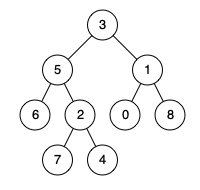# 236. Lowest Common Ancestor of a Binary Tree / Medium

Given a binary tree, find the lowest common ancestor (LCA) of two given nodes in the tree.

According to the definition of LCA on Wikipedia: “The lowest common ancestor is defined between two nodes p and q as the lowest node in T that has both p and q as descendants (where we allow a node to be a descendant of itself).”

## Example 1:Input: root = [3,5,1,6,2,0,8,null,null,7,4], p = 5, q = 1
utput: 3
Explanation: The LCA of nodes 5 and 1 is 3.

## Example 2:Input: root = [3,5,1,6,2,0,8,null,null,7,4], p = 5, q = 4
Output: 5
Explanation: The LCA of nodes 5 and 4 is 5, since a node can be a descendant of itself according to the LCA definition.

## Example 3:

Input: root = [1,2], p = 1, q = 2
Output: 1

## Comstraints

• The number of nodes in the tree is in the range [2, 10^5].
• -10^9 <= Node.val <= 10^9
• All Node.val are unique.
• p != q
• p and q will exist in the tree.

# Solution: Recursive

## 思路## 效能

### Complexity

• Time Complexity: O(N)
• Space Complexity: O(N)

### LeetCode Result

• Runtime: 24 ms
• Memory Usage: 14.2 MB